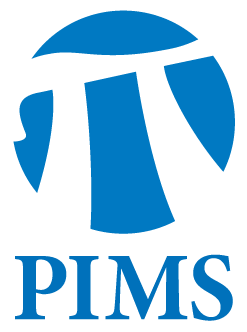## Topology Seminar: Galo Higuera Rojo

• Date: 03/07/2012
• Time: 15:00
Lecturer(s):
Galo HJiguera Rojo, UBC
Location:

University of British Columbia

Topic:

Spaces of homomorphisms and commuting matrices

Description:

Abstract:

Given a lie group G and a finitely generated group P we can give a topology to the set of group homomorphisms Hom(P,G) as a subset of G^k. There has been an increasing interest in understanding these spaces, and particularly their connected components,  for their relevance in bundle theory. In particular when P=Z^k the space Hom(Z^k,G) is identified with the set of commuting k-tuples in G. We will present some of the generalities of these spaces and a possible systematic approach to their study. Then we will use that approach applied to the particular case when G=O(n), the group of orthogonal matrices, and compute the number of components of Hom(Z^k,O(n)).

Other Information:

Location: WMAX 216